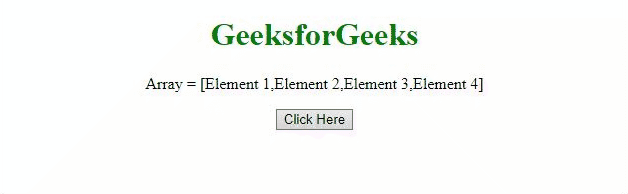# Alternatives of push() method in JavaScript

The task is to perform push operation without using the push() method with the help of JavaScript. There are two approaches that are discussed below.

Approach 1: Use the length property to insert the element at the end of the array.

• Example: This example implements the above approach.

 ` ` `<``html``> ` `    ``<``head``> ` `        ``<``title``> ` `            ``Alternatives of push() method in Javascript ` `        `` ` `    `` ` ` `  `    ``<``body` `style``=``"text-align: center;"``> ` `        ``<``h1` `style``=``"color: green;"``> ` `            ``GeeksforGeeks ` `        `` ` `        ``<``p` `id``=``"GFG_UP"``> ` `        ``<``button` `onclick``=``"myGFG()"``> ` `            ``Click Here ` `        `` ` `        ``<``p` `id``=``"GFG_DOWN"``> ` `   ``<``script``> ` `     ``var arr = ["Element 1", "Element 2",  ` `                 ``"Element 3", "Element 4"]; ` `     ``var up = document.getElementById("GFG_UP"); ` `      ``up.innerHTML = "Array = [" + arr + "]"; ` `     ``var element = "Element x"; ` `     ``var down = document.getElementById("GFG_DOWN"); ` `     ``function myGFG() { ` `      ``arr[arr.length] = element; ` `    ``down.innerHTML = "Elements of array = [" + arr + "]"; ` `            ``} ` `        `` ` `    `` ` ` `

• Output:Approach 2 Use the [] notation to insert the element at the end of array.

• Example: This example implements the above approach.

 ` ` `<``html``> ` `    ``<``head``> ` `        ``<``title``> ` `            ``Alternatives of push() method in Javascript ` `        `` ` `    `` ` ` `  `    ``<``body` `style``=``"text-align: center;"``> ` `        ``<``h1` `style``=``"color: green;"``> ` `            ``GeeksforGeeks ` `        `` ` `        ``<``p` `id``=``"GFG_UP"``> ` `        ``<``button` `onclick``=``"myGFG()"``> ` `            ``Click Here ` `        `` ` `        ``<``p` `id``=``"GFG_DOWN"``> ` `  ``<``script``> ` `   ``var arr = ["Element 1", "Element 2",  ` `              ``"Element 3", "Element 4"]; ` `   ``var up = document.getElementById("GFG_UP"); ` `   ``up.innerHTML = "Array = [" + arr + "]"; ` `   ``var element = "Element x"; ` `   ``var down = document.getElementById("GFG_DOWN"); ` `   ``function myGFG() { ` `   ``arr = [arr, element]; ` `  ``down.innerHTML = "Elements of array = [" + arr + "]"; ` `            ``} ` `        `` ` `    `` ` ` `

• Output:My Personal Notes arrow_drop_upCheck out this Author's contributed articles.

If you like GeeksforGeeks and would like to contribute, you can also write an article using contribute.geeksforgeeks.org or mail your article to contribute@geeksforgeeks.org. See your article appearing on the GeeksforGeeks main page and help other Geeks.

Please Improve this article if you find anything incorrect by clicking on the "Improve Article" button below.